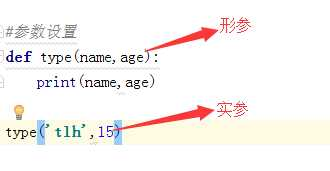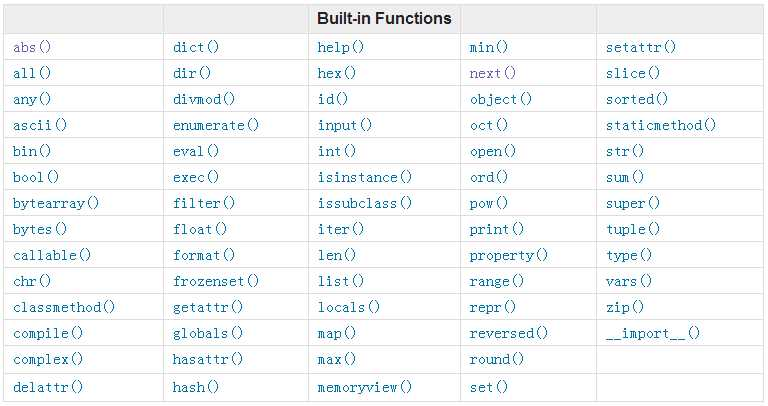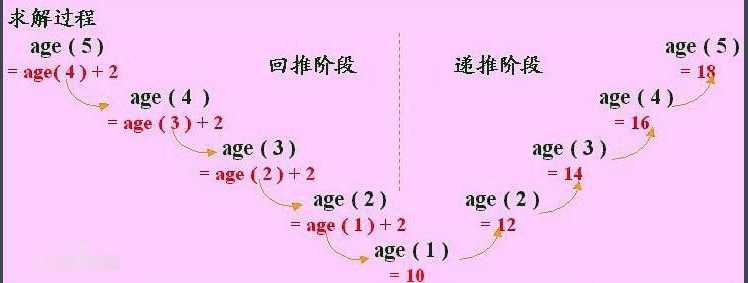# Python基础之函数

一、背景

在学习函数之前，一直遵循：面向过程编程，即：根据业务逻辑从上到下实现功能，其往往用一长段代码来实现指定功能，开发过程中最常见的操作就是粘贴复制，也就是将之前实现的代码块复制到现需功能处，如下：

```while True：
if cpu利用率 > 90%:
#发送邮件提醒
连接邮箱服务器
发送邮件
关闭连接

if 硬盘使用空间 > 90%:
#发送邮件提醒
连接邮箱服务器
发送邮件
关闭连接

if 内存占用 > 80%:
#发送邮件提醒
连接邮箱服务器
发送邮件
关闭连接```

```def 发送邮件(内容)
#发送邮件提醒
连接邮箱服务器
发送邮件
关闭连接

while True：

if cpu利用率 > 90%:
发送邮件(‘CPU报警‘)

if 硬盘使用空间 > 90%:
发送邮件(‘硬盘报警‘)

if 内存占用 > 80%:```

• 函数式：将某功能代码封装到函数中，日后便无需重复编写，仅调用函数即可
• 面向对象：对函数进行分类和封装，让开发“更快更好更强...”

### 二、函数的定义和使用

#### 定义: 函数是指将一组语句的集合通过一个名字(函数名)封装起来，要想执行这个函数，只需调用其函数名即可

1. 减少重复代码
2. 使程序变的可扩展
3. 使程序变得易维护
```def 函数名(参数):

...
函数体
...
返回值```

• def：表示函数的关键字
• 函数名：函数的名称，日后根据函数名调用函数
• 函数体：函数中进行一系列的逻辑计算，如：发送邮件、计算出 [11,22,38,888,2]中的最大数等...
• 参数：为函数体提供数据
• 返回值：当函数执行完毕后，可以给调用者返回数据。

### 1、返回值

函数是一个功能块，该功能到底执行成功与否，需要通过返回值来告知调用者。

1.函数在执行过程中只要遇到return语句，就会停止执行并返回结果，所以也可以理解为 return 语句代表着函数的结束。

2.return 只有在python中如果没有写return，python会自动给你返回一个None。但其他语言不会。

```def 发送短信():

发送短信的代码...

if 发送成功:
return True
else:
return False

while True:

# 每次执行发送短信函数，都会将返回值自动赋值给result
# 之后，可以根据result来写日志，或重发等操作

result = 发送短信()
if result == False:
记录日志，短信发送失败..```

### 2、函数的参数

形参：变量只有在被调用时才分配内存单元，在调用结束时，即刻释放所分配的内存单元。因此，形参只在函数内部有效。函数调用结束返回主调用函数后则不能再使用该形参变量

实参：可以是常量、变量、表达式、函数等，无论实参是何种类型的量，在进行函数调用时，它们都必须有确定的值，以便把这些值传送给形参。因此应预先用赋值，输入等办法使参数获得确定值使用参数可以简化函数重复性。```def CPU报警邮件()
#发送邮件提醒
连接邮箱服务器
发送邮件
关闭连接

def 硬盘报警邮件()
#发送邮件提醒
连接邮箱服务器
发送邮件
关闭连接

def 内存报警邮件()
#发送邮件提醒
连接邮箱服务器
发送邮件
关闭连接

while True：

if cpu利用率 > 90%:
CPU报警邮件（）

if 硬盘使用空间 > 90%:
硬盘报警邮件（）

if 内存占用 > 80%:
内存报警邮件（）``````def 发送邮件(邮件内容)

#发送邮件提醒
连接邮箱服务器
发送邮件
关闭连接

while True：

if cpu利用率 > 90%:
发送邮件("CPU报警了。")

if 硬盘使用空间 > 90%:
发送邮件("硬盘报警了。")

if 内存占用 > 80%:
发送邮件("内存报警了。")```

• 普通参数
• 默认参数
• 动态参数```# ######### 定义函数 #########

# name 叫做函数func的形式参数，简称：形参
def func(name):
print name

# ######### 执行函数 #########
#  ‘wupeiqi‘ 叫做函数func的实际参数，简称：实参
func(‘wupeiqi‘)``````def func(name, age = 18):

print "%s:%s" %(name,age)

# 写入参数
func(‘wupeiqi‘, 19)
# 使用默认参数
func(‘alex‘)```def func(name, age):
print("%s:%s" %(name,age))
#接收参数(在传参过程中，如果没有指定参数，形式参数会按照你实际参数的顺序去传。)
func(‘wupeiqi‘,19 )
# 指定参数(在传参过程中，我们可以通过指定参数改变顺序。)
func(age=19,name=‘wupeiqi‘)``````def func(*args):
print(args,type(args))

# 执行方式一
li = [11,2,2,3,3,4,54]
func(li，‘12‘)  #会把li当成*args中其中一个元素，

# 执行方式二
li = [11,2,2,3,3,4,54]
func(*li)    #加上*后会把列表中的所有元素转化为元组到*args中``````def func(**kwargs):

print args

#执行形式必须使用‘指定参数‘来传参数，否则报错

# 执行方式一
func(name＝‘wupeiqi‘,age=18)

# 执行方式二
li = {‘name‘:‘wupeiqi‘, age:18, ‘gender‘:‘male‘}
func(**li)

#执行方式三
dic = {‘name‘:‘wupeiqi‘, age:18, ‘gender‘:‘male‘}
func(kk=dic)```
```#元祖形式的封装到*args中，字典形式的封装到**kwars。
# 默认:大家都写成*args、**kwars
def func(*args,**kwars):
print(args)
print(kwars)
func(11,22,33,k1=‘k1‘,k2=‘k2‘)

```#######格式化#######
1、第一种格式化数字用%d、字符用%s
a=‘tlh‘
b=123
print(‘number is %d,str is %s‘%(b,a))
2、第二种格式化format

s="i am {0}, age{1}".format(*["alex",18])
print(s)

s="i am {name}, age{age}".format(name=‘tlh‘,age=18])
dic={‘name‘:‘tlh‘,‘arg‘:18}
s="i am {name}, age{age}".format(**dic)
print(s)```

### 3、函数注意点：```def f1(a1,a2):
return a1+a2
def f1(a1,a2):
return a1*a2
ret=f1(8,8)
print(ret)``````########函数参数的传递#######
def f1(a1):
a1.append(999)
li=[11,22,333]
#函数如果传递
#1、引用(li在内存中的地址)，那么在运行函数时就是把li本身传递到函数中进行处理。
#2、值(li在内存中重新复制一份)，那么在运行函数时就是li的复制，li本身没有发生变化。
f1(li)
#但在函数中都是用的引用，改变都是li本身的值。
print(li)``````######作用域######
#作用域以函数分割
def f1():
name=‘tlh‘
def f2():
print(name)
#全局变量,在所有的作用域都可读
NAME=‘tlh‘
def f1():
age=18
print(name,age)
f1()
#局部变量
NAME=‘tlh‘
def f2():
#如果函数内定义了和全局变量一样的变量，优先使用局部变量。
name=‘aaaa‘
age=17
print(name,age)
f2()
#对全局变量修改(global)
def f3():
global name=‘aaaa‘
age=17
print(name,age)
f3()
#对特殊的全局变量进行添加,如：列表和字典，但不可赋值(NAME=‘aa‘).
NAME=[‘tlh‘,‘aa‘,‘bbb‘]
def f4():
NAME.append(‘dd‘)
f4()
print(name)```

例如：```def login(username,password):
"""
注释：登录判断
"""
f =open("db",‘r‘)
for line in f:
return True
return False
"""
注释:注册用户
"""
f = open("db", ‘r‘)
f.write(temp)
f.close()
def main():
"""
注释：执行的主程序
"""
t =input("1:登录 2:注册")
if t==‘1‘:
user=input(‘请输入用户名‘)
pwd=input(‘请输入密码‘)
if r:
print(‘登录成功‘)
else:
print(‘登录失败‘)
if t==‘2‘:
user = input(‘请输入用户名‘)
pwd = input(‘请输入密码‘)
register(user,pwd)
main()```

### 三、内置函数abs() 返回数字的绝对值

int（）将字符串或者小数转换成整数

str（）将其他类型转换是字符型

len（X）返回X的长度。

print（）输出

type（X）返回X的数据类型

open（f）打开一个文件f并返回文件类型的对象。

dir()  查看该对象的所有函数

### 四、其他

主要是一些对方函数的补充，如：递归和迭代，全局变量和局部变量等。

### 1、三元运算

学习条件运算时，对于简单的 if else 语句，可以使用三元运算来表示，即：

```# 普通条件语句
if 1 == 1:
name = ‘wupeiqi‘
else:
name = ‘alex‘

# 三元运算
name = ‘wupeiqi‘ if 1 == 1 else ‘alex‘```

### 2、lambda表达式

对于简单的函数，也存在一种简便的表示方式，即：lambda表达式

```# ###################### 普通函数 ######################
# 定义函数（普通方式）
def func(arg):
return arg + 1

# 执行函数
result = func(123)

# ###################### lambda ######################

# 定义函数（lambda表达式）
my_lambda = lambda arg : arg + 1 #默认return，只能简单的赋值。

# 执行函数
result = my_lambda(123)```

### 3、发送邮件实例```import smtplib
from email.mime.text import MIMEText

msg = MIMEText(‘邮件内容‘, ‘plain‘, ‘utf-8‘)
msg[‘Subject‘] = "主题"

server = smtplib.SMTP("smtp.126.com", 25)
server.sendmail(‘[email protected]‘, [‘[email protected]‘,], msg.as_string())
server.quit()```

### 4、递归和迭代1. 必须有一个明确的结束条件

2. 每次进入更深一层递归时，问题规模相比上次递归都应有所减少

3. 递归效率不高，递归层次过多会导致栈溢出（在计算机中，函数调用是通过栈（stack）这种数据结构实现的，每当进入一个函数调用，栈就会加一层栈帧，每当函数返回，栈就会减一层栈帧。由于栈的大小不是无限的，所以，递归调用的次数过多，会导致栈溢出）

```#程序的结果就是如此
def num(n):
print(n)
if n/2 >1:
res = num(n/2)
print(‘N:‘,n)
return n
num(10)```示例代码

迭代```#求1到5的和就是迭代，range函数默认从0到5.
sum_i = 0
for i in range(1,5+1):
print(i)
sum_i=sum_i+i
print(sum_i)``````#普通循环
sum_i = [11,‘aa‘,‘bbb‘,44]
for i in range(len(sum_i )):
print(sum_i[i])```

### 5、全局变量和局部变量

```#定义全局变量
a=2
def number(b):
num = a+b
return num
number_num=number(5)
print(number_num)```

```#定义局部变量，局部变量只能在函数内使用
name = ‘yes‘
def type(name):
print(‘查看name的值：‘,name)
name = ‘我改变了‘
print(‘当前的name值：‘,name)
type(name)
print(‘看外面改变了吗？‘,name)```
global语句(全局变量申明)

```#使用global可以改变全局变量的值
name = ‘yes‘
def type():
global name
name = ‘我改变了‘
print(‘当前的name值：‘,name)
type()
print(‘看外面改变了吗？‘,name)```

Python基础之函数

(0)
(0)